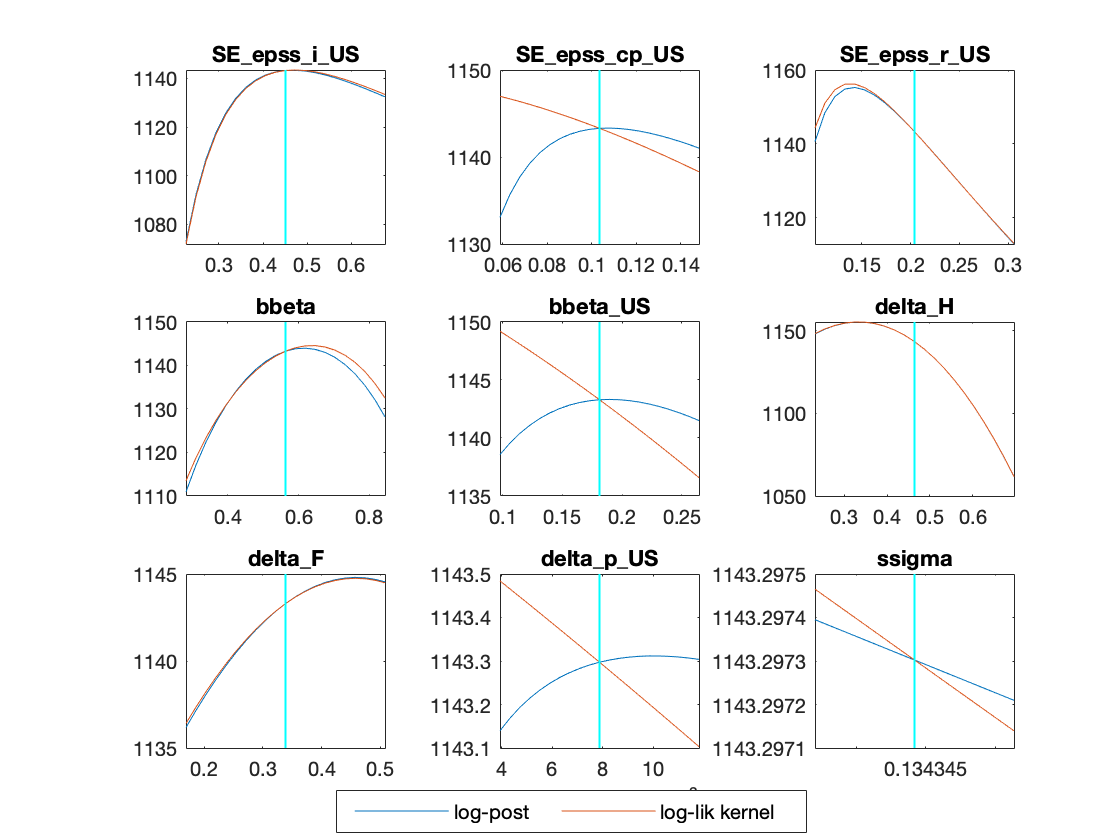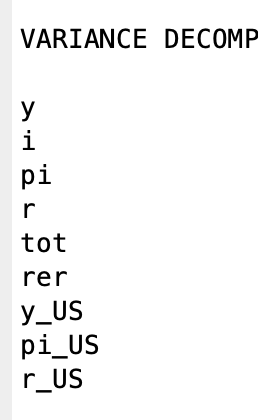# Blanchard & Kahn conditions are not satisfied: no stable equilibrium & The Jacobian of the static model is singular

@TerribleStudent In case of equation (42) above the data is mean 0 by construction. Why is it not in your case?
@Shells What is the error message your are getting in in Matlab 2021?

Sorry, you’re correct, both rates have mean zero (8.081644e-20 to be precise).

I’ve solved most mode_check plots by adjusting the data for inflation (as specified in the manual) and respecified the mean and SD for some priors. It seems the model doesn’t solve/work for some specific values of the parameters (even in stoch_simul) … is this common?

That depends on the reason. It is very common for some parameter vectors to not satisfy the Blanchard-Kahn conditions. But the red dots can be caused by other pathological issues. Setting

``````options_.debug=1;
``````

I get these plots currentlyand the following messages:
"Estimation::marginal density: Let me try again.
Estimation::marginal density: There’s probably a problem with the modified harmonic mean estimator.

ESTIMATION RESULTS

Log data density is -Inf."

and lastly:

“DsgeSmoother: Switching to univariate filter. This is usually due to co-integration in diffuse filter,
otherwise it may be a sign of stochastic singularity.”

How to interpret it?

">> options_.debug=1

options_ =

struct with fields:

``````                                           datafile: 'dataset1'
dirname: 'model'
dataset: [1×1 struct]
verbosity: 1
terminal_condition: 0
rplottype: 0
smpl: 0
dynatol: [1×1 struct]
slowc: 1
timing: 0
gstep: [2×1 double]
scalv: 1
debug: 1
initval_file: 0
schur_vec_tol: 1.0000e-11
qz_criterium: []
qz_zero_threshold: 1.0000e-06
lyapunov_complex_threshold: 1.0000e-15
solve_tolf: 6.0555e-06
solve_tolx: 3.6669e-11
dr_display_tol: 1.0000e-06
minimal_workspace: 0
dp: [1×1 struct]
simul: [1×1 struct]
mode_check: [1×1 struct]
huge_number: 10000000
jacobian_flag: 1
ParamSubSet: 'None'
dsge_var: 0
dsge_varlag: 4
bvar_replic: 2000
bvar_prior_tau: 3
bvar_prior_decay: 0.5000
bvar_prior_lambda: 5
bvar_prior_mu: 2
bvar_prior_omega: 1
bvar_prior_flat: 0
bvar_prior_train: 0
bvar: [1×1 struct]
optim_opt: []
gmhmaxlik: [1×1 struct]
nointeractive: 0
graphics: [1×1 struct]
graph_format: 'eps'
nodisplay: 0
nograph: 0
no_graph: [1×1 struct]
XTick: []
XTickLabel: []
console_mode: 0
irf: 40
impulse_responses: [1×1 struct]
zero_moments_tolerance: 1.0000e-10
relative_irf: 0
ar: 5
hp_filter: 0
one_sided_hp_filter: 0
filtered_theoretical_moments_grid: 512
nodecomposition: 0
nomoments: 0
nocorr: 0
periods: 0
noprint: 0
SpectralDensity: [1×1 struct]
nofunctions: 0
bandpass: [1×1 struct]
irf_opt: [1×1 struct]
ep: [1×1 struct]
bnlms: [1×1 struct]
particle: [1×1 struct]
rwgmh: [1×1 struct]
TeX: 0
xls_sheet: 1
xls_range: ''
prior_draws: 10000
sampling_draws: 500
forecast: 0
forecasts: [1×1 struct]
conditional_forecast: [1×1 struct]
linear: 1
stack_solve_algo: 0
markowitz: 0.5000
minimal_solving_periods: 1
endogenous_terminal_period: 0
no_homotopy: 0
pfwee: [1×1 struct]
order: 1
pruning: 0
solve_algo: 4
replic: 50
simul_replic: 1
drop: 100
aim_solver: 0
k_order_solver: 0
partial_information: 0
ACES_solver: 0
conditional_variance_decomposition: []
ramsey_policy: 0
instruments: {}
timeless: 0
ramsey: [1×1 struct]
initial_period: NaN
no_init_estimation_check_first_obs: 0
Harvey_scale_factor: 10
heteroskedastic_filter: 0
MaxNumberOfBytes: 100000000
MaximumNumberOfMegaBytes: 111
analytic_derivation: 0
analytic_derivation_mode: 0
bayesian_irf: 1
bayesian_th_moments: 0
diffuse_filter: 1
filtered_vars: 0
smoothed_state_uncertainty: 0
first_obs: 1
nobs: 106
kalman_algo: 0
fast_kalman_filter: 0
kalman_tol: 1.0000e-10
kalman: [1×1 struct]
diffuse_kalman_tol: 1.0000e-06
use_univariate_filters_if_singularity_is_detected: 1
riccati_tol: 1.0000e-06
lik_algo: 1
lik_init: 3
logdata: 0
loglinear: 0
linear_approximation: 0
mh_conf_sig: 0.9000
prior_interval: 0.9000
mh_drop: 0.5000
mh_jscale: 0.1802
mh_tune_jscale: [1×1 struct]
mh_init_scale: 0.4000
mh_initialize_from_previous_mcmc: [1×1 struct]
mh_nblck: 2
mh_recover: 0
mh_replic: 20000
recursive_estimation_restart: 0
MCMC_jumping_covariance: 'hessian'
use_calibration_initialization: 0
endo_vars_for_moment_computations_in_estimation: []
occbin: [1×1 struct]
silent_optimizer: 0
prior_restrictions: [1×1 struct]
mode_compute: 6
mode_file: ''
moments_varendo: 0
nk: []
noconstant: 0
nodiagnostic: 0
mh_posterior_mode_estimation: 0
prefilter: 0
presample: 0
prior_trunc: 1.0000e-10
smoother: 0
smoother_redux: 0
posterior_max_subsample_draws: 1200
sub_draws: 1200
ME_plot_tol: 1.0000e-06
use_mh_covariance_matrix: 0
posterior_sampler_options: [1×1 struct]
trace_plot_ma: 200
mh_autocorrelation_function_size: 30
plot_priors: 1
cova_compute: 1
parallel: 0
parallel_info: [1×1 struct]
number_of_grid_points_for_kde: 512
conditional_variance_decomposition_dates: [1 5 9 13 17 37 77 117 157 197]
filter_covariance: 0
filter_decomposition: 0
selected_variables_only: 0
contemporaneous_correlation: 0
initialize_estimated_parameters_with_the_prior_mode: 0
estimation: [1×1 struct]
homotopy_mode: 0
homotopy_steps: 1
homotopy_force_continue: 0
rescale_prediction_error_covariance: 0
hessian: [1×1 struct]
csminwel: [1×1 struct]
newrat: [1×1 struct]
simplex: [1×1 struct]
cmaes: [1×1 struct]
simpsa: [1×1 struct]
solveopt: [1×1 struct]
saopt: [1×1 struct]
particleswarm: [1×1 struct]
prior_mc: 20000
prior_analysis_endo_var_list: {}
block: 0
use_dll: 0
bytecode: 0
sylvester_fp: 0
sylvester_fixed_point_tol: 1.0000e-12
lyapunov_fp: 0
lyapunov_db: 0
lyapunov_srs: 0
lyapunov_fixed_point_tol: 1.0000e-10
lyapunov_doubling_tol: 1.0000e-16
dr_cycle_reduction: 0
dr_cycle_reduction_tol: 1.0000e-07
dr_logarithmic_reduction: 0
dr_logarithmic_reduction_tol: 1.0000e-12
dr_logarithmic_reduction_maxiter: 100
initial_date: [1×1 dates]
discretionary_policy: 0
discretionary_tol: 1.0000e-07
parameter_set: []
use_shock_groups: ''
shock_decomp: [1×1 struct]
plot_shock_decomp: [1×1 struct]
nonlinear_filter: []
ms: [1×1 struct]
graph_save_formats: [1×1 struct]
endogenous_prior: 0
endogenous_prior_restrictions: [1×1 struct]
osr: [1×1 struct]
gpu: 0
convergence: [1×1 struct]
marginal_data_density: [1×1 struct]
lmmcp: [1×1 struct]
lcppath: [1×1 struct]
mcppath: [1×1 struct]
figures: [1×1 struct]
varobs_id: [41 42 43 44 45 46 47 48 49 50]
pac: [1×1 struct]
DynareRandomStreams: [1×1 struct]
varobs: {10×1 cell}
varlist: {8×1 cell}
number_of_observed_variables: 10
B: 1200
``````

"

There are 21 eigenvalue(s) larger than 1 in modulus
for 22 forward-looking variable(s)

The rank condition ISN’T verified!

Error using print_info (line 32)
Blanchard & Kahn conditions are not satisfied: indeterminacy.

Error in stoch_simul (line 107)
print_info(info, options_.noprint, options_);

Error in US_IC3_v2.driver (line 1185)
[info, oo_, options_, M_] = stoch_simul(M_, options_, oo_, var_list_);

Error in dynare (line 293)
evalin(‘base’,[fname ‘.driver’]) ;

@Shells So you are saying the model ran without the BK violations in Matlab 2017, but not in 2021? If that is the case, please provide the file.
@TerribleStudent The `options_.debug=1;` will lead to additional output during mode_check of red dots appear. This is not the case here. It seems rather that you are missing

``````prior_trunc=0;
``````

It was running fine in matlab 2017 (without the BK errors) yes. After the matlab update, I have tried too many different versions of the mod file however, so I am not sure anymore what the exact original version was. Except of course I am still getting the BK-related errors. The latest mod file of course is attached in my very first comment/question above - just not sure if it is the exact same one that was giving me no errors in matlab 2017. Sorry!

I’m getting more red dots with prior_trunc=0 which I have trouble solving. This is my estimation settings

``````estimation(datafile=dataset1, first_obs=1, mh_replic=10000, mh_nblocks=2, mh_drop=0.5, mh_jscale=0.4, mode_compute=6, mode_check, bayesian_irf, diffuse_filter, prior_trunc=0) y c i n pi r tot rer;
``````

any suggestions or ideas? I’m attaching my code/data if anyone have time to check. Thank you.
dataset1.mat (8.7 KB)
model.mod (7.7 KB)

The red dots mostly indicate that your model is very close to the boundary to instability.

Can that be solved by using upper- and lower bounds on the priors?

Not really. You would be replacing one corner solution with another bound, i.e. an implicit prior with an explicit one. Rather, you would need to make the prior more informative, or even better try to understand why the data prefers something close to instability.

Thank you for all your help, the only issue currently is that some of the Brooks and Gelman (1998) plots doesn’t converge, I guess thats also related to the priors, I’ll keep investigating.

I do have one question not related to the part above: Is it okey to report in a paper/thesis the “Variance decomposition (in percent)” obtained from the stoch_simul and bayesian irf from the estimation command? Or shouldn’t one mix them?
I’m interest in the estimation of the parameters, bayesian irf and variance decomposition, but what I’ve read on the forum it’s hard to interpret the VD from the estimation command.
I’ll then use the following commands

``````estimation(datafile=dataset1, first_obs=1, mh_replic=20000, mh_nblocks=2, mh_drop=0.5, mh_jscale=0.3, mode_compute=6, mode_check, bayesian_irf, diffuse_filter, prior_trunc=0) y c i n pi r tot rer;
stoch_simul(order=1, irf=0) y i pi r tot rer y_US i_US pi_US r_US;
``````

Edit; One of my variables doesn’t show up in the VD in stoch_simul, namely i_US what could be the reason?Sincerely,
TerribleStudent

1. Both objects (the mean variance decomposition over the posterior parameters from `estimation` and the variance decomposition for the mean parameter draw from `stoch_simul`) measure different things. Thus, you should not mix them but rather use the one that is most suitable for your application.
2. DId you check whether the variance of `i_US` is neither 0 nor infinity?
1. So I shouldn’t mix the bayesian irf with the variance decomposition obtained from the stoch_simul, rather choose between bayesian irf and mean variance decomposition or irf and VD from stoch_simul with the estimated parameters?

2. There is some problem with mean, SD and variance, what could possible be the reason for this?

EIGENVALUES:
Modulus Real Imaginary

``````   4.344e-17       -4.344e-17                0
9.928e-17       -9.928e-17                0
0.2701           0.2701                0
0.2799           0.2799                0
0.4071           0.3452           0.2158
0.4071           0.3452          -0.2158
0.5398           0.3356           0.4228
0.5398           0.3356          -0.4228
0.5541           0.4626            0.305
0.5541           0.4626           -0.305
0.595           0.4721           0.3622
0.595           0.4721          -0.3622
0.7503           0.7503                0
0.8              0.8                0
0.8              0.8                0
0.8              0.8                0
0.8              0.8                0
0.8              0.8                0
0.8              0.8                0
0.8              0.8                0
0.8              0.8                0
0.8              0.8                0
0.8              0.8                0
0.8              0.8                0
0.8              0.8                0
0.8359           0.8336          0.06173
0.8359           0.8336         -0.06173
0.976            0.976                0
0.9966           0.9966                0
1                1                0
1.045            1.045                0
1.192            1.163           0.2594
1.192            1.163          -0.2594
1.194            1.194                0
1.382            1.382                0
1.603            1.603                0
2.029            2.029                0
2.041            2.041                0
2.817            2.461            1.371
2.817            2.461           -1.371
3.677e+16       -3.677e+16                0
1.065e+17        1.065e+17                0
1.172e+17        1.172e+17                0
1.998e+17       -1.998e+17                0
3.027e+18       -3.027e+18                0
``````

There are 15 eigenvalue(s) larger than 1 in modulus
for 15 forward-looking variable(s)

The rank condition is verified.

1. Sorry, I meant you wanted to combine the same object (variance decomposition) from `stoch_simul` and `estimation`. What you suggest should be fine as long as you clearly outline it. But note that a referee may prefer you to be fully Bayesian.
2. I outlined the two reasons: `i_US` may be constant so that the variance is 0 or it may have a unit root so that the variance is infinite.
1. Yes, thats what I thought.

2. So when I run the model_diagnostics(M_,options_,oo_) I get the following

the i_US equation is specified as

``````i_US=(1/(1+bbeta_US))*i_US(-1)+(bbeta_US/(1+bbeta_US))*i_US(+1)+(1/(chi_bis_US*(1+bbeta_US)))*(q_US+eps_i_US);
``````

which is the same as for the domestic i variable equation. However, when I remove either `(1/(1+bbeta_US))*i_US(-1)` or `(bbeta_US/(1+bbeta_US))*i_US(+1)` I get the following in the model_diagnosticsand i_US shows up in both the theoretical moments and VD etc.

So my question are:

1. why would excluding the `(1/(1+bbeta_US))*i_US(-1)` part from the foreign investment equation solve the problem when it works for the domestic? Also this is a common investment equation in the literature where both i(-1) and i(+1) is present, for example Smets and Wouters.

2. also this means I change some key equations which means its not consistent with the log-linearization process by pen and pencil, how should one proceed?

3. this indicate that (at least) 2 equations in this published paper is falsely.